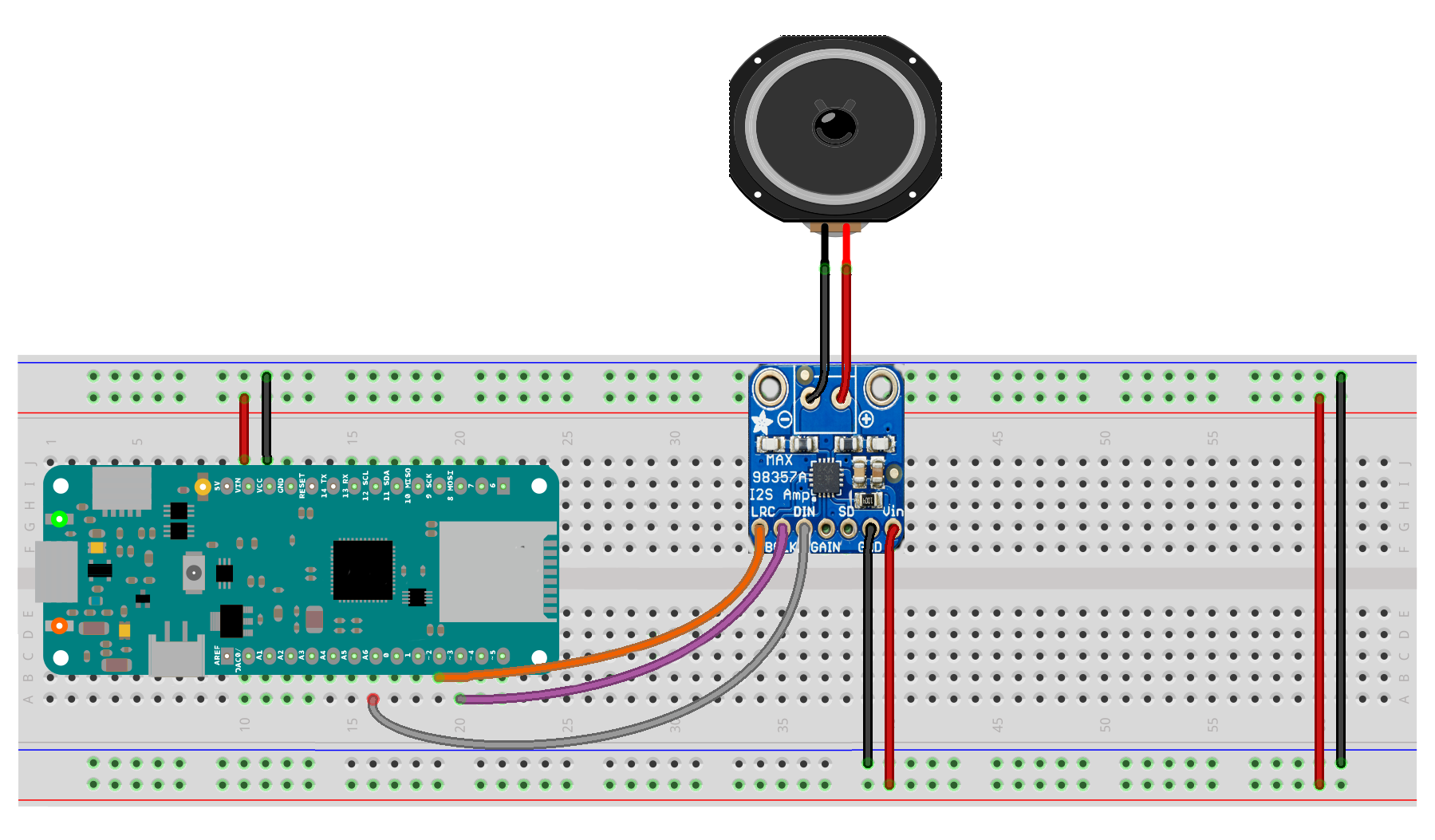## SimpleTone

This example shows you how to generate a simple tone using a SAMD21 based board (MKRZero, MKR1000 or Zero) and an I2S DAC like the adafruit MAX98357A.

### Circuit

To run this example you simply have to connect the board and the I2S DAC using the I2S bus as shown in the image. The image is for MKRZero; you find the proper pins for Zero and MKR1000 at the beginning of the sketch, in the comments.image developed using Fritzing. For more circuit examples, see the Fritzing project page

### Code

/*
This example generates a square wave based tone at a specified frequency
and sample rate. Then outputs the data using the I2S interface to a
MAX08357 I2S Amp Breakout board.

Circuit:
* Arduino/Genuino Zero, MKR family and Nano 33 IoT
* MAX08357:
* GND connected GND
* VIN connected 5V
* LRC connected to pin 0 (Zero) or 3 (MKR) or A2 (Nano)
* BCLK connected to pin 1 (Zero) or 2 (MKR) or A3 (Nano)
* DIN connected to pin 9 (Zero) or A6 (MKR) or 4 (Nano)

created 17 November 2016
by Sandeep Mistry
*/

#include <I2S.h>

const int frequency = 440; // frequency of square wave in Hz
const int amplitude = 500; // amplitude of square wave
const int sampleRate = 8000; // sample rate in Hz

const int halfWavelength = (sampleRate / frequency); // half wavelength of square wave

short sample = amplitude; // current sample value
int count = 0;

void setup() {
Serial.begin(9600);
Serial.println("I2S simple tone");

// start I2S at the sample rate with 16-bits per sample
if (!I2S.begin(I2S_PHILIPS_MODE, sampleRate, 16)) {
Serial.println("Failed to initialize I2S!");
while (1); // do nothing
}
}

void loop() {
if (count % halfWavelength == 0) {
// invert the sample every half wavelength count multiple to generate square wave
sample = -1 * sample;
}

// write the same sample twice, once for left and once for the right channel
I2S.write(sample);
I2S.write(sample);

// increment the counter for the next sample
count++;
}